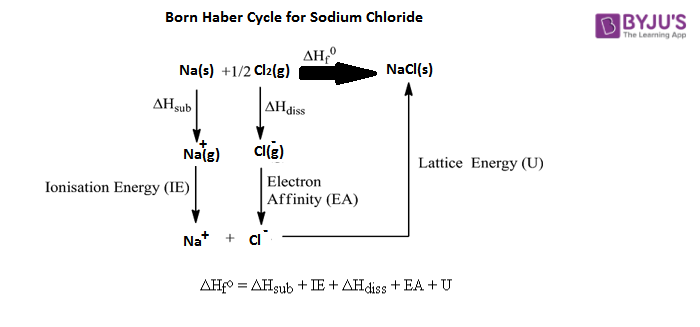Checkout JEE MAINS 2022 Question Paper Analysis : Checkout JEE MAINS 2022 Question Paper Analysis :

# Born Haber Cycle

## What is Born Haber Cycle?

The Born–Haber cycle is a method of analysing reaction energy. Two German scientists Fritz Haber and Max Born developed it in the year 1919 and it was therefore named after them. It explains and helps in understanding the formation of ionic compounds. It is primarily used to calculate lattice energy as it cannot be measured directly.

## What is Lattice Enthalpy?

The change in enthalpy that occurs during the formation of an ionic compound from the exothermic process. It can also be defined as the energy required to break an ionic compound into the endothermic process.

## What is Hess’s Law?

Hess’s Law states that when a reactant is converted to a product, then the change in enthalpy is the same irrespective of the reaction taking place in one step or series of steps. Born Haber Cycle uses Hess’s law to calculate lattice enthalpy.

Some important concepts to understand before implementing Born-Haber Cycle are discussed below:

• Ionisation Energy or ionisation potential – The energy required to remove an electron from a neutral atom with external energy is called ionisation energy. Therefore, it will always hold a positive value. Ionisation energy increases from left to right in the periodic table but decreases from top to bottom.
• Electron Affinity – The energy liberated when an electron is added to a neutral atom. Generally, the energy released is a negative value, therefore, while calculating the lattice energy, subtract the electron affinity. Electron affinity increases from left to right in the periodic table but decreases from top to bottom.
• Dissociation energy – The energy required to break a compound is called dissociation energy.
• The heat of formation – There is a change in energy when an element forms a compound. This energy is called the heat of formation.
• Sublimation energy – The energy required to change a solid state to a gas, with the help of input energy. Therefore, it is a positive value.

## How to use Born Haber Cycle?

• Step 1: Determine the energy of the non-metal and metal in their elemental form. From this subtract the heat of formation. The value obtained is the energy of the ionic solid, which is used to determine the lattice energy.
• Step 2: The elements involved in the reactions should be in their gaseous forms. Add the changes in enthalpy to change one of the elements to its gaseous form, and repeat the same with the other elements.
• Step 3: Since metals in nature occur as a single atom, no dissociation energy is added to this element. But, many non-metals occur as polyatomic species such as Cl exists as Cl2 in its elemental state. Therefore, the energy required to transform Cl2 into 2Cl, atoms are needed to be added to the value obtained in Step 2.
• Step 4: Change the metal and non-metal into their ionic forms from their ionic solid. Add the ionisation energy of the metal for the value obtained from Step 3. Further, subtract electron affinity of the non-metal from the previous value.
• Step 5: In this step, the metal and non-metal will combine to form the ionic solid, causing a release of energy. This energy is called the lattice energy. The final value for the lattice energy is the difference between the values of Step 1 and Step 4.Test your knowledge on born haber cycle